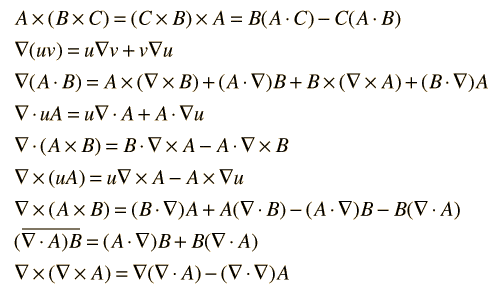# Simplify the proof of different vector calculus identities

Is there a way to simplify the proof of different vecot calculus identities, such as grad of f*g, which is expandable. And also curl of the curl of a field. Is there a more convenient way to go about proving these relations than to go through the long calculations of actually performing the curl and grad etc? I know nothing about geometric algebra, but is that a good way?Im talking about all these identities, is there a branch of mathematics that simplifies the proofs of these, and lets me avoid expending the vectors and del operators?

robphy
Homework Helper
Gold Member
Fredrik
Staff Emeritus
•